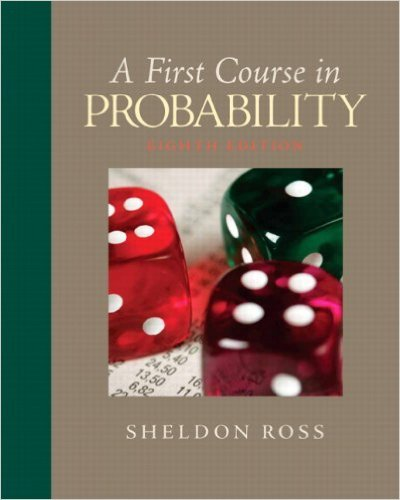×

×

# Solutions for Chapter 2: Axioms of Probability## Full solutions for First Course in Probability | 8th Edition

ISBN: 9780136033134Solutions for Chapter 2: Axioms of Probability

Solutions for Chapter 2
4 5 0 404 Reviews
20
2
##### ISBN: 9780136033134

Summary of Chapter 2: Axioms of Probability

First Course in Probability was written by and is associated to the ISBN: 9780136033134. Since 56 problems in chapter 2: Axioms of Probability have been answered, more than 26541 students have viewed full step-by-step solutions from this chapter. This expansive textbook survival guide covers the following chapters and their solutions. This textbook survival guide was created for the textbook: First Course in Probability, edition: 8. Chapter 2: Axioms of Probability includes 56 full step-by-step solutions.

Key Statistics Terms and definitions covered in this textbook
• -error (or -risk)

In hypothesis testing, an error incurred by rejecting a null hypothesis when it is actually true (also called a type I error).

• Assignable cause

The portion of the variability in a set of observations that can be traced to speciic causes, such as operators, materials, or equipment. Also called a special cause.

• Attribute control chart

Any control chart for a discrete random variable. See Variables control chart.

• Bimodal distribution.

A distribution with two modes

• Bivariate normal distribution

The joint distribution of two normal random variables

• Center line

A horizontal line on a control chart at the value that estimates the mean of the statistic plotted on the chart. See Control chart.

• Central tendency

The tendency of data to cluster around some value. Central tendency is usually expressed by a measure of location such as the mean, median, or mode.

• Chance cause

The portion of the variability in a set of observations that is due to only random forces and which cannot be traced to speciic sources, such as operators, materials, or equipment. Also called a common cause.

• Components of variance

The individual components of the total variance that are attributable to speciic sources. This usually refers to the individual variance components arising from a random or mixed model analysis of variance.

• Conditional mean

The mean of the conditional probability distribution of a random variable.

• Crossed factors

Another name for factors that are arranged in a factorial experiment.

• Design matrix

A matrix that provides the tests that are to be conducted in an experiment.

• Discrete distribution

A probability distribution for a discrete random variable

• Enumerative study

A study in which a sample from a population is used to make inference to the population. See Analytic study

• Estimate (or point estimate)

The numerical value of a point estimator.

• Estimator (or point estimator)

A procedure for producing an estimate of a parameter of interest. An estimator is usually a function of only sample data values, and when these data values are available, it results in an estimate of the parameter of interest.

• Exponential random variable

A series of tests in which changes are made to the system under study

• Fractional factorial experiment

A type of factorial experiment in which not all possible treatment combinations are run. This is usually done to reduce the size of an experiment with several factors.

• Gamma function

A function used in the probability density function of a gamma random variable that can be considered to extend factorials

• Generating function

A function that is used to determine properties of the probability distribution of a random variable. See Moment-generating function